# canvas实践小实例 —— 扇形

//将原点移到100,100的位置
ctx.translate(100, 100);
//画一个圆弧
ctx.arc(0,0,100,30*Math.PI/180, 60*Math.PI/180);
ctx.stroke();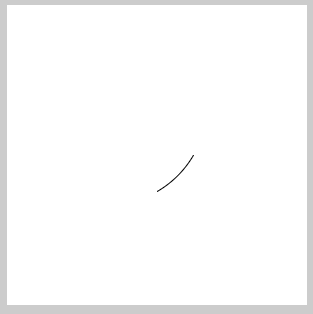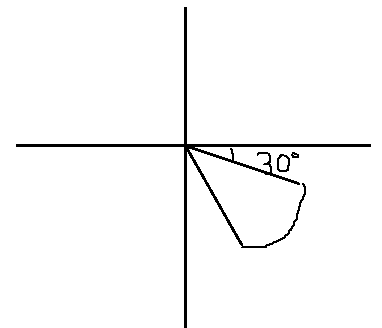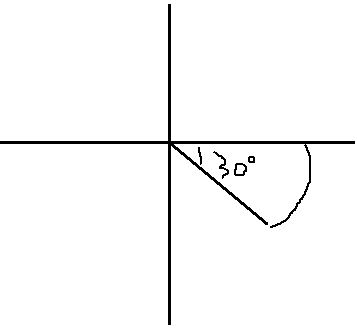//圆弧
ctx.save();
ctx.translate(100, 100);
ctx.arc(0,0,100,0, 30*Math.PI/180);
ctx.restore();
//第一条线
ctx.save();
ctx.moveTo(100,100);
ctx.lineTo(200,100);
ctx.restore();
//第二条线
ctx.save();
ctx.translate(100, 100);
ctx.moveTo(0,0);
ctx.rotate(30*Math.PI/180);
ctx.lineTo(100,0);
ctx.stroke();
ctx.restore();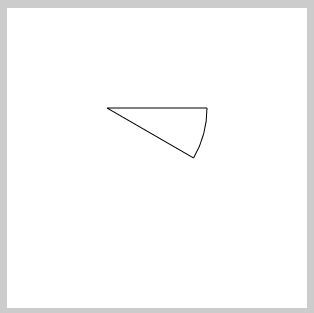//将原点设置100,100位置
ctx.translate(100,100);
//原点在100,100，则圆心设为0,0 ——> 100,100的位置
ctx.arc(0,0,100,30*Math.PI/180,60*Math.PI/180);
//save(),restore()是为了防止角度旋转的污染
ctx.save();
ctx.rotate(30*Math.PI/180);
ctx.moveTo(0,0);
ctx.lineTo(100,0);
ctx.restore();
ctx.rotate(60*Math.PI/180);
ctx.moveTo(0,0);
ctx.lineTo(100,0);
ctx.stroke();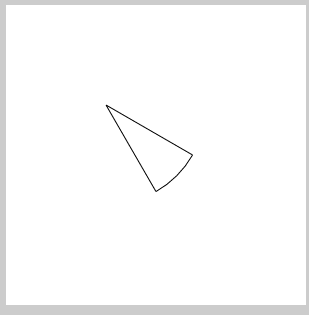//将原点设置100,100位置
ctx.translate(100,100);
//原点在100,100，则圆心设为0,0 ——> 100,100的位置
ctx.arc(0,0,100,30*Math.PI/180,60*Math.PI/180);
//save(),restore()是为了防止角度旋转的污染
ctx.save();
ctx.rotate(30*Math.PI/180);
ctx.moveTo(0,0);
ctx.lineTo(100,0);
ctx.restore();
ctx.rotate(60*Math.PI/180);
ctx.lineTo(100,0);
ctx.stroke();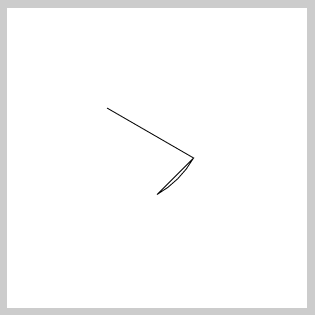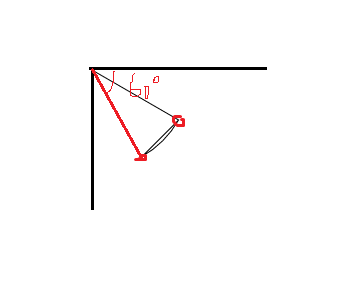//将原点设置100,100位置
ctx.translate(100,100);
//原点在100,100，则圆心设为0,0 ——> 100,100的位置
ctx.arc(0,0,100,30*Math.PI/180,60*Math.PI/180);
//save(),restore()是为了防止角度旋转的污染
ctx.save();
ctx.rotate(30*Math.PI/180);
ctx.moveTo(100,0);
ctx.lineTo(0,0);
ctx.restore();
ctx.rotate(60*Math.PI/180);
ctx.lineTo(100,0);
ctx.stroke();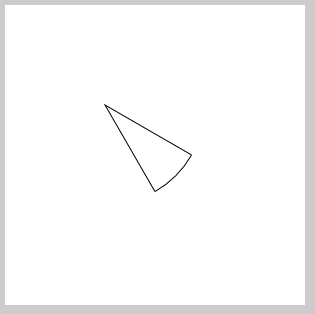//将原点设置100,100位置
ctx.translate(100,100);
//原点在100,100，则圆心设为0,0 ——> 100,100的位置
ctx.arc(0,0,100,30*Math.PI/180,60*Math.PI/180);
//以圆弧终点为起点画直线
ctx.lineTo(0,0);
ctx.rotate(30*Math.PI/180);
//以0,0为起点画直线
ctx.lineTo(100,0);
ctx.stroke();CanvasRenderingContext2D.prototype.sector = function(x,y,r,sDeg,eDeg){
this.save();
this.translate(x,y);
this.beginPath();
this.arc(0,0,r,sDeg*Math.PI/180,eDeg*Math.PI/180);
this.save();
this.rotate(sDeg*Math.PI/180);
this.moveTo(r,0);
this.lineTo(0,0);
this.restore();
this.rotate(eDeg*Math.PI/180);
this.lineTo(r,0);
this.restore();
return this;
}
ctx.sector(100,100,100,30,60).stroke();
ctx.sector(100,100,100,90,120).fill();
ctx.sector(100,100,100,160,180).stroke();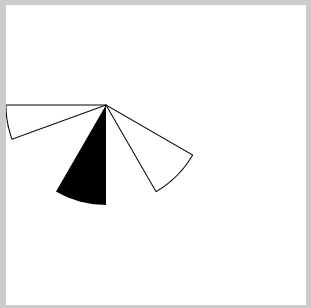CanvasRenderingContext2D.prototype.sector = function(x,y,r,sDeg,eDeg){
this.save();
this.translate(x,y);
this.beginPath();
this.arc(0,0,r,sDeg*Math.PI/180,eDeg*Math.PI/180);
this.lineTo(0,0);
this.rotate(sDeg*Math.PI/180);
this.lineTo(r,0);
this.restore();
return this;
}
ctx.sector(100,100,100,30,60).stroke();
ctx.sector(100,100,100,90,120).fill();
ctx.sector(100,100,100,160,180).stroke();ctx.beginPath();
//定义起点
ctx.moveTo(100,100);
//以起点为圆心，画一个半径为100的圆弧
ctx.arc(100,100,100,30*Math.PI/180, 60*Math.PI/180);
ctx.closePath();
ctx.stroke();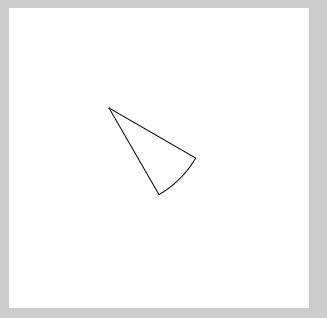CanvasRenderingContext2D.prototype.sector = function(x,y,r,angle1,angle2){
this.save();
this.beginPath();
this.moveTo(x,y);
this.arc(x,y,r,angle1*Math.PI/180,angle2*Math.PI/180,false);
this.closePath();
this.restore();
return this;
}
ctx.sector(100,100,100,30,60).stroke();
ctx.sector(100,100,100,90,120).fill();
ctx.sector(100,100,100,160,180).stroke();CanvasRenderingContext2D.prototype.sector = function(x,y,r,angle1,angle2){
this.save();
this.beginPath();
this.moveTo(x,y);
this.arc(x,y,r,angle1*Math.PI/180,angle2*Math.PI/180,false);
this.closePath();
this.restore();
return this;
}
ctx.fillStyle = 'red';
ctx.sector(200,200,100,30,150).fill();
ctx.fillStyle = 'green';
ctx.sector(200,200,100,150,270).fill();
ctx.fillStyle = 'blue';
ctx.sector(200,200,100,270,390).fill();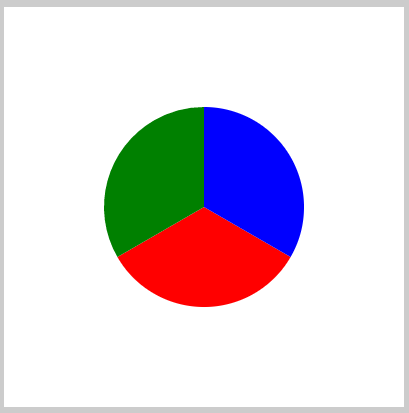CanvasRenderingContext2D.prototype.sector = function(x,y,r,angle1,angle2){
this.save();
this.beginPath();
this.moveTo(x,y);
this.arc(x,y,r,angle1*Math.PI/180,angle2*Math.PI/180,false);
this.closePath();
this.restore();
return this;
}

var angle = 0;
var timer = null;
ctx.fillStyle = 'green';
setInterval(function(){
angle+=5;
ctx.sector(200,200,100,0,angle).fill();
if(angle == 360){
clearInterval(timer);
}
},200);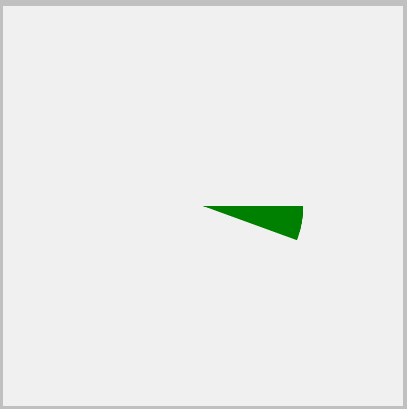CanvasRenderingContext2D.prototype.sector = function(x,y,r,angle1,angle2){
this.save();
this.beginPath();
this.moveTo(x,y);
this.arc(x,y,r,angle1*Math.PI/180,angle2*Math.PI/180,false);
this.closePath();
this.restore();
return this;
}
ctx.fillStyle = 'red';
ctx.sector(200,200,100,30,150).fill();
ctx.fillStyle = 'green';
ctx.sector(200,200,100,150,270).fill();
ctx.fillStyle = 'blue';
ctx.sector(200,200,100,270,390).fill();
ctx.fillStyle = '#fff';
ctx.sector(200,200,80,0,360).fill();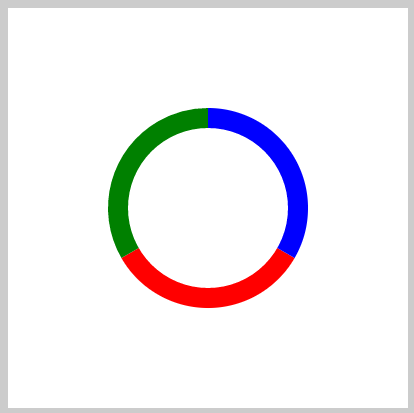CanvasRenderingContext2D.prototype.sector = function(x,y,r,angle1,angle2){
this.save();
this.beginPath();
this.moveTo(x,y);
this.arc(x,y,r,angle1*Math.PI/180,angle2*Math.PI/180,false);
this.closePath();
this.restore();
return this;
}

var angle = 0;
var timer = null;
setInterval(function(){
angle+=5;
ctx.fillStyle = 'green';
ctx.sector(200,200,100,0,angle).fill();
ctx.fillStyle = '#fff';
ctx.sector(200,200,80,0,360).fill();
if(angle == 360){
clearInterval(timer);
}

},200);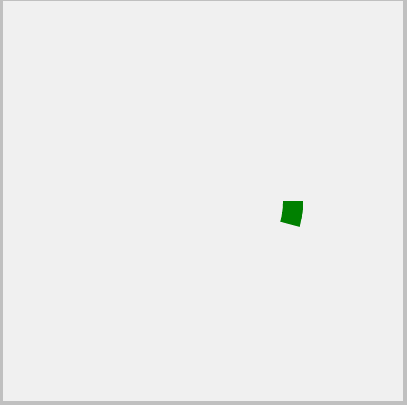©️2019 CSDN 皮肤主题: 黑客帝国 设计师: 上身试试